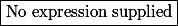# Matematické Fórum

Nevíte-li si rady s jakýmkoliv matematickým problémem, toto místo je pro vás jako dělané.

Nástěnka
!! 17.06.2018 (Jel.) Khanova škola zve nadšence ke spolupráci na překladech návodů pro učitele a rodiče.
! 04.11.2016 (Jel.) Čtete, prosím, před vložení dotazu, děkuji!
17.01.2016 (Jel.) Rok 2016 s novými a novějšími krystaly od kolegy Pavla!
17.01.2016 (Jel.) Nabídka knih z oborů matematiky, fyziky, chemie
23.10.2013 (Jel.) Zkuste před zadáním dotazu použít některý z online-nástrojů, konzultovat použití můžete v sekci CAS.

Nejste přihlášen(a). Přihlásit

## #1 24. 12. 2018 06:33 — Editoval stuart clark (24. 12. 2018 06:33)

stuart clarkPříspěvky: 1007
Reputace:

### real solution

The number of solution  of the equation$\displaystyle \int^{|x|}_{0}\bigg((t^2-1)\cdot \ln(t)\bigg)dt=\frac{5}{6}|x|.$ for all$x\in \mathbb{R}_{0}$

Offline

## #2 24. 12. 2018 16:56

jardofprPříspěvky: 1231
Reputace:   87

### Re: real solution

hi ↑ stuart clark:

one solution should be$x=0$

I believe that as one way to proceed you could find antiderivative to the left side of the equation
and then rewrite the equation as$f(x)=0$
what you only need then is first and second derivative of$f$ on positive half of the real line
(as$f$ will be even function) getting 2 more positive real solutions, ultimately getting total of 5 real solutions

in more detail if needed

Offline

## #3 05. 01. 2019 10:04

stuart clarkPříspěvky: 1007
Reputace:

### Re: real solution

Thanks ↑ jardofpr:

Offline